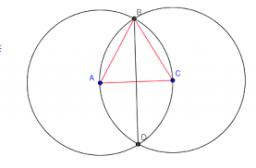# Intersect 6042

Two circles with straight radii of 58 mm intersect at two points. Their common string is 80 mm long. What is the distance of the centers of these circles?

x =  84 mm

### Step-by-step explanation:Did you find an error or inaccuracy? Feel free to write us. Thank you!

Showing 1 comment:
Cjinct
Unless I am missing something, triangle ABC is an equilateral triangle since all three sides are radii and therefore are congruent.  If I am correct, you only need to know the length of the radii to answer the question ... AC = 58 cm and BD = 58(root 3) cm.

Tips for related online calculators